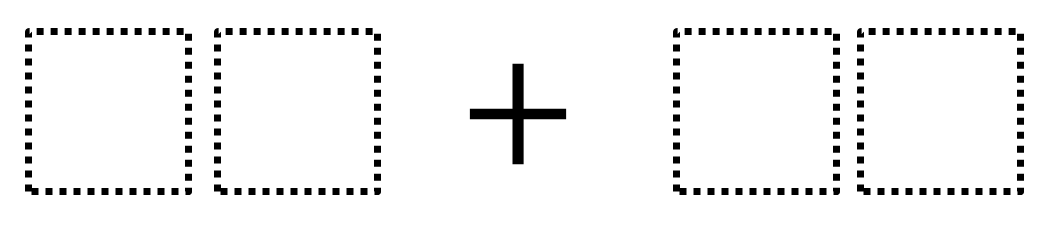Directions: Using the digits 1 to 9 at most one time each, fill in the boxes to make the smallest (or largest) sum.### Hint

In the two-digit number, what does the digit on the left represent?  What does the digit on the right represent?

97 + 86 is one answer for the largest sum.  13 + 24 is the smallest sum.

Source: Robert Kaplinsky

## Time Twister

Directions: Using the digits 0 to 9, at most one time each, create three different …

1.My second grade class loves coming up with variations on this. My personal favorite so far is what is the largest sum you can make with the biggest difference between the two numbers? I also like what is the largest/smallest you can make with only even or odd numbers?

2.ANTHONY FITZPATRICK

96+87 for largest and 14+23 for smallest . Since you are only moving a “one”, the value of the sum remains the same

3.40+60=100

4.97+86

5.98+76

6.lasr :13+24=37
high:97+86=183

7.8.9.19 + 43

10.Smallest :13+24=37
highest:97+86=183

11.12 + 34 =46

98 + 76 = 174

12.small 38+50 = 88
large 70+ 40 = 110

13.14.97+86
13+24

15.smallest-15+27
largest-45+36

16.16+82=98

17.12+36=48

no repeating numbers and all numbers are less than 9

18.19.Largest 97+86
Smallest 13+24

20.LARGE 87+96
SMALL 1+2